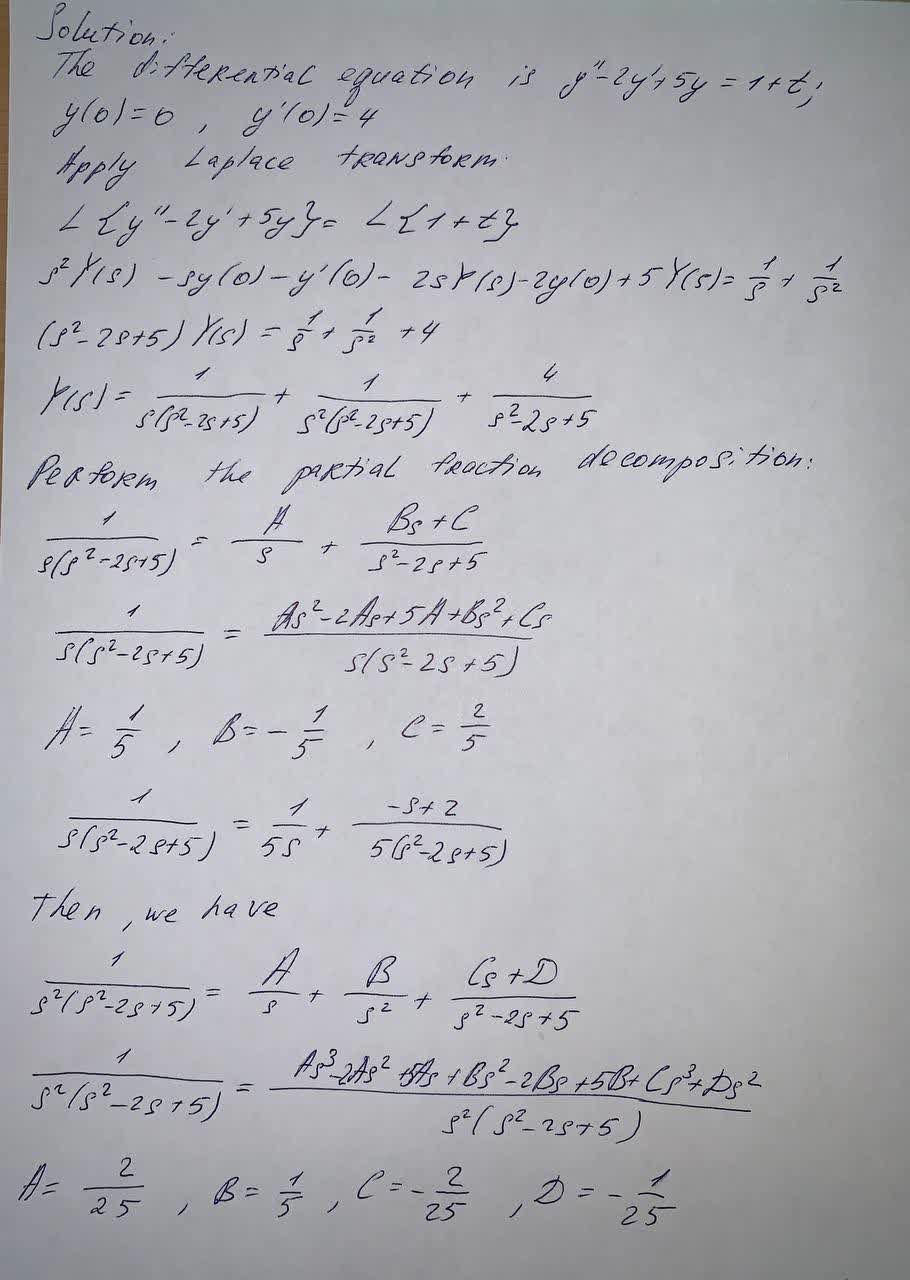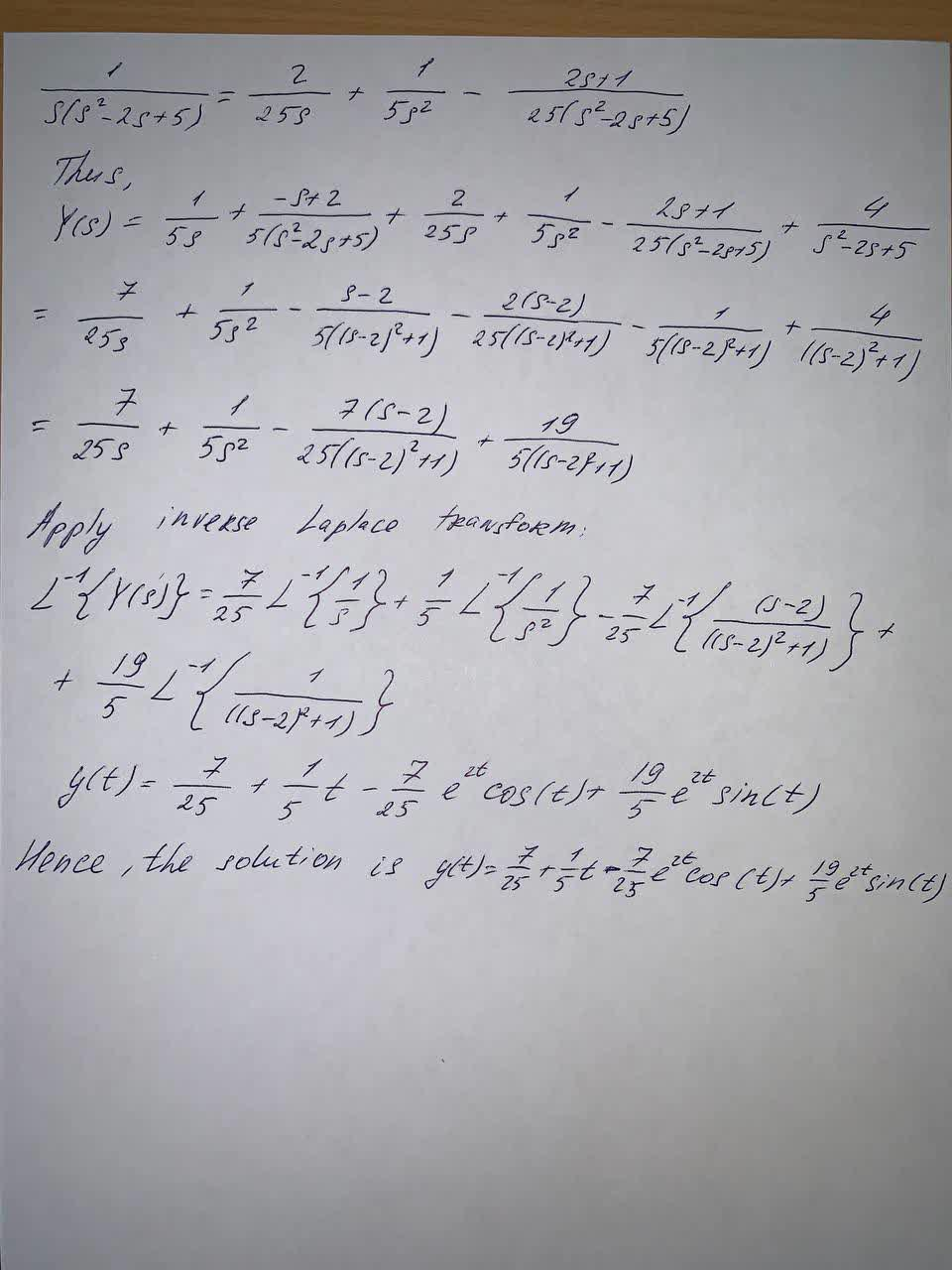# Use Laplace transform to solve the following initial value problem:y"-2y'+5y=1+t , y(0)=0 , y'(0)=4Clifland 2021-09-24 Answered

Use Laplace transform to solve the following initial value problem:
${y}^{″}+5y=1+t,y\left(0\right)=0,{y}^{\prime }\left(0\right)=4$
A)$\frac{7}{25}{e}^{t}\mathrm{cos}\left(2t\right)+\frac{21}{10}{e}^{t}\mathrm{sin}\left(2t\right)$
B) $\frac{7}{2}+\frac{t}{5}-\frac{t}{25}{e}^{t}\mathrm{cos}\left(2t\right)+\frac{21}{10}{e}^{t}\mathrm{sin}\left(2t\right)$
C) $\frac{7}{25}+\frac{t}{5}$
D) $\frac{7}{25}+\frac{t}{5}-\frac{7}{25}{e}^{t}\mathrm{cos}\left(2t\right)+\frac{21}{10}{e}^{t}\mathrm{sin}\left(2t\right)$
E) non of the above
F) $\frac{7}{25}+\frac{t}{5}-\frac{7}{5}{e}^{t}\mathrm{cos}\left(2t\right)+\frac{21}{10}{e}^{t}\mathrm{sin}\left(2t\right)$

You can still ask an expert for help

## Want to know more about Laplace transform?

• Questions are typically answered in as fast as 30 minutes

Solve your problem for the price of one coffee

• Math expert for every subject
• Pay only if we can solve itescumantsu
Now we have